# Algebra 3/4 : Simplify Functions

## Example Questions

### Example Question #1 : Algebra 3/4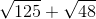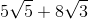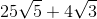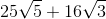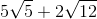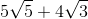Explanation:

To simplify the radical expression look at the factors under each radical.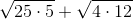Recall that 25 and 4 are perfect squares.From here 12 can be factored further.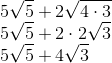### Example Question #1 : Simplify Radical Expressions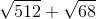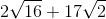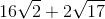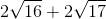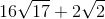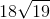Explanation:

To simplify the radical expression look at the factors under each radical.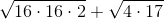Recall that 16 and 4 are perfect squares.### Example Question #2 : Simplify FunctionsExplanation:

To simplify the radical expression look at the factors under each radical.Recall that 25 and 4 are perfect squares.From here 12 can be factored further.### Example Question #1 : Simplify Radical ExpressionsExplanation:

To simplify the radical expression look at the factors under each radical.Recall that 16 and 4 are perfect squares.### All Algebra 3/4 Resources